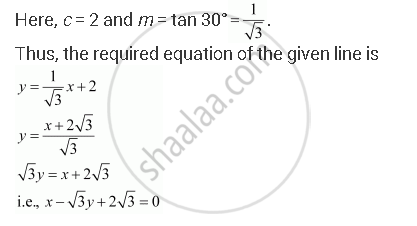CBSE (Arts) Class 11CBSE
Share

# Find the Equation of the Line Which Intersects the Y-axis at a Distance of 2 Units Above the Origin and Makes an Angle of 30° with the Positive Direction of the X-axis. - CBSE (Arts) Class 11 - Mathematics

ConceptVarious Forms of the Equation of a Line

#### Question

Find the equation of the line which intersects the y-axis at a distance of 2 units above the origin and makes an angle of 30° with the positive direction of the x-axis.

#### Solution

It is known that if a line with slope makes y-intercept c, then the equation of the line is given as

mx + cIs there an error in this question or solution?

#### Video TutorialsVIEW ALL 

Solution Find the Equation of the Line Which Intersects the Y-axis at a Distance of 2 Units Above the Origin and Makes an Angle of 30° with the Positive Direction of the X-axis. Concept: Various Forms of the Equation of a Line.
S